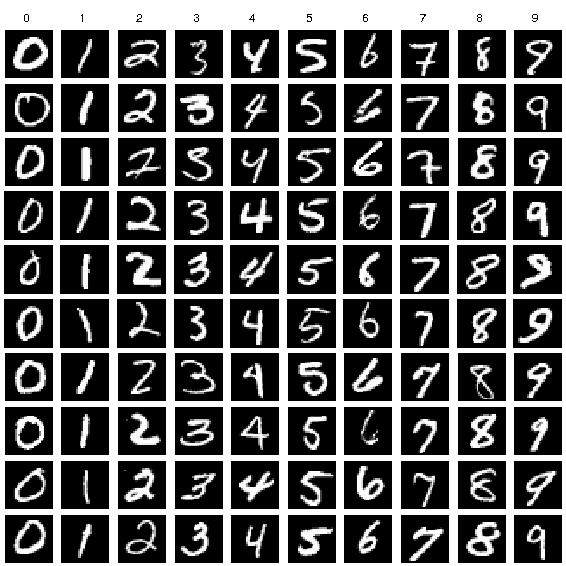# Handwritten Digit Recognition MNIST Dataset

Get FREE domain for 1st year and build your brand new siteMINIST dataset is widely used dataset in machine learning for handwritten recognition, image classification and many more. The MNIST dataset is short form for the Modified National Institute of Standards and Technology dataset.

It is a dataset of 60,000 small square 28×28 pixel grayscale images of handwritten single digits between 0 and 9. Which is quiet incredible to explore and analyse.

Handwritten recognition project specifically performs the classification a given image of a handwritten digit into one of 10 classes representing integer values from 0 to 9, including both 0 and 9.

It is a mostly used and profoundly understood dataset and, for the most part, is “solved.” Top-performing models are deep learning convolutional neural networks that achieve a classification accuracy of above 99%, with an error rate between 0.4 %and 0.2% on the hold out test dataset.

### Algorithmic Steps

1. Import all the required libaries
3.Analysing Baseline Model with Multi-Layer Perceptrons
3.Building Simple Convolutional Neural Network for MNIST
4.Building Larger Convolutional Neural Network for MNIST

### Implementations

First step for any machine learning programming is loading the required libraries and importing the necessary modules as follows:

``````
from keras.datasets import mnist
from keras.models import Sequential
from keras.layers import Dense
from keras.layers import Dropout
from keras.layers import Flatten
from keras.layers.convolutional import Conv2D
from keras.layers.convolutional import MaxPooling2D
from keras.utils import np_utils

``````

Now after all the modules have been loaded, we then load our test set and training set respectively, as follows. Note the syntax of loading the test set data and the training set data from MNIST dataset available in keras. We then have to reshape our training set and test set.

``````# load data
(X_train, y_train), (X_test, y_test) = mnist.load_data()
# reshape to be [samples][width][height][channels]
X_train = X_train.reshape(X_train.shape, 28, 28, 1).astype('float32')
X_test = X_test.reshape(X_test.shape, 28, 28, 1).astype('float32')

``````

Next step is normalising the inputs. Normalization is a technique often applied as part of data preparation for machine learning. The goal of normalization is to change the values of numeric columns in the dataset to use a common scale, without distorting differences in the ranges of values or losing information. Further we categorise our data. Categorical Data is the data that generally takes a limited number of possible values. Also, the data in the category need not be numerical, it can be textual in nature. All machine learning models are some kind of mathematical model that need numbers to work with.That categorical data is defined as variables with a finite set of label values. That most machine learning algorithms require numerical input and output variables. That an integer and one hot encoding is used to convert categorical data to integer data.

``````# normalize inputs from 0-255 to 0-1
X_train = X_train / 255
X_test = X_test / 255
# one hot encode outputs
y_train = np_utils.to_categorical(y_train)
y_test = np_utils.to_categorical(y_test)
num_classes = y_test.shape
``````

A baseline is a method that uses heuristics, simple summary statistics, randomness, or machine learning to create predictions for a dataset. You can use these predictions to measure the baseline's performance (e.g., accuracy)-- this metric will then become what you compare any other machine learning algorithm against. A simple baseline model can be created as follows.

``````def baseline_model():
# create model
model = Sequential()
model.add(Conv2D(32, (5, 5), input_shape=(1, 28, 28), activation='relu'))
# Compile model
return model
...
# build the model
model = baseline_model()
# Fit the model
model.fit(X_train, y_train, validation_data=(X_test, y_test), epochs=10, batch_size=200, verbose=2)
``````

Model Evaluation is an integral part of the model development process. It helps to find the best model that represents our data and how well the chosen model will work in the future. To avoid overfitting, both methods use a test set (not seen by the model) to evaluate model performance.

``````# Final evaluation of the model
scores = model.evaluate(X_test, y_test, verbose=0)
print("CNN Error: %.2f%%" % (100-scores*100))
``````

### Building Simple CNN Model for MNIST

Putting all the above concepts together, here is a full-fledged Convolutional neural network model for building of MNIST dataset.

``````from keras.datasets import mnist
from keras.models import Sequential
from keras.layers import Dense
from keras.layers import Dropout
from keras.layers import Flatten
from keras.layers.convolutional import Conv2D
from keras.layers.convolutional import MaxPooling2D
from keras.utils import np_utils
(X_train, y_train), (X_test, y_test) = mnist.load_data()
X_train = X_train.reshape((X_train.shape, 28, 28, 1)).astype('float32')
X_test = X_test.reshape((X_test.shape, 28, 28, 1)).astype('float32')
X_train = X_train / 255
X_test = X_test / 255
y_train = np_utils.to_categorical(y_train)
y_test = np_utils.to_categorical(y_test)
num_classes = y_test.shape
def baseline_model():

model = Sequential()
model.add(Conv2D(32, (5, 5), input_shape=(28, 28, 1), activation='relu'))

return model
model = baseline_model()
model.fit(X_train, y_train, validation_data=(X_test, y_test), epochs=10, batch_size=200)
scores = model.evaluate(X_test, y_test, verbose=0)
print("CNN Error: %.2f%%" % (100-scores*100))
``````

A example of Images in MNIST dataset is as follows:### Use Cases of MNIST Dataset

1. MNIST dataset is used widely for handwrittern digit classifier.
2. It is the supporting base for handwritting, signature recognisation.
3. MNIST dataset is also used for image classifiers dataset analysis.
4. MNIST Dataset is an intergal part of Date predictions from pieces of texts in coorporate world.
5. MNIST dataset is also used for predicting the students percentages from their resumes in order to check their qualifying level.